# For the stop bracket shown, locate the x coordinate of the center of gravity.

Question-AnswerCategory: Engineering MechanicsFor the stop bracket shown, locate the x coordinate of the center of gravity.

For the stop bracket shown, locate the x coordinate of the center of gravity.Step: 1

Divide the stop bracket into sections as follows:Step: 2

Assume that the bracket is homogeneous. Accordingly, the center of gravity is to coincide with the centroid of the volume.
Write the equation for x-coordinate of center as follows:……. (1)Here,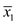is the x-coordinate centroid of section 1,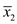is the x-coordinate centroid of section 2,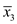is the x-coordinate centroid of section 3,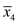is the x-coordinate centroid of section 4,is the volume of section 1,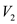is the volume of section 2,is the volume of section 3 and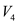is the volume of section 4.

Step: 3

Calculate the x-coordinate centroids of each individual section and corresponding volumes and tabulate the values as follows:

Volume no:

Volume ‘V’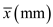I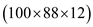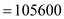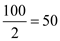5280000

II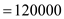6000000?

III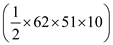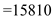616590

IV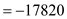– 1389960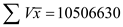Step: 4

Calculate the x-coordinate of center for the given bracket.
Substituteforandfor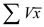in equation (1).Therefore, the x-coordinate of center for the given bracket is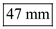.